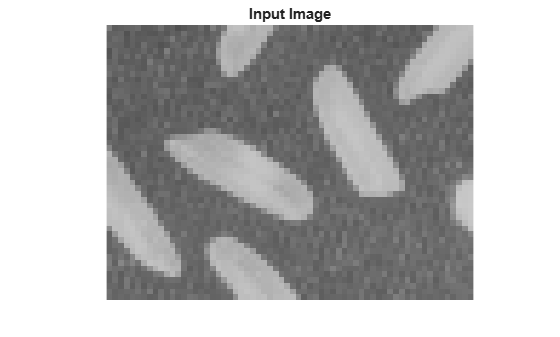# visionhdl.ImageStatistics

Mean, variance, and standard deviation

## Description

The `visionhdl.ImageStatistics` System object™ calculates the mean, variance, and standard deviation of streaming video data. The object performs each calculation over all pixels in the input region of interest (ROI) and implements the calculations by using hardware-efficient algorithms.

• To change the size and dimensions of the ROI, you can manipulate the input video stream control signals. See Regions of Interest.

• The number of valid pixels in the input image affect the accuracy of the mean approximation. To avoid approximation errors, use an image that contains fewer than 64 pixels, a multiple of 64 pixels up to 642 pixels, a multiple of 4096 pixels up to 643 pixels, or a multiple of 643 pixels up to 644 pixels. For details of the mean approximation, see Algorithm.

• The object calculates statistics over frames up to 644 (16,777,216) pixels in size.

To calculate mean, variance, and standard deviation:

1. Create the `visionhdl.ImageStatistics` object and set its properties.

2. Call the object with arguments, as if it were a function.

## Creation

### Syntax

``stats = visionhdl.ImageStatistics(Name,Value)``

### Description

example

````stats = visionhdl.ImageStatistics(Name,Value)` returns a System object that calculates statistics from an input image region. Set properties using one or more name-value pairs. Enclose each property name in single quotes.For example, when you configure the object like this, the object returns mean and standard deviation, but not variance:stats = visionhdl.ImageStatistics('mean',true, ... 'variance',false, ... 'stdDev',true) ```

## Properties

expand all

Unless otherwise indicated, properties are nontunable, which means you cannot change their values after calling the object. Objects lock when you call them, and the `release` function unlocks them.

If a property is tunable, you can change its value at any time.

If you set this property to `false`, the object does not return the mean.

If you set this property to `false`, the object does not return the variance.

If you set this property to `false`, the object does not return the standard deviation.

## Usage

### Syntax

``[mean,variance,stddeviation,validout] = stats(pixelin,ctrlin)``

### Description

example

````[mean,variance,stddeviation,validout] = stats(pixelin,ctrlin)` incorporates one pixel value, `pixelIn`, into ongoing calculations of video frame statistics. The control signals associated with each pixel, `ctrlin`, indicate the frame boundaries. When `validout` is `true`, the output values of `mean`, `variance`, and `stddeviation` represent the statistics for the most recent input frame completed. The number of statistics returned depends on the object property settings.```

This object uses a streaming pixel interface with a structure for frame control signals. The interface enables the object to operate independently of image size and format and connect with other Vision HDL Toolbox™ objects. The object accepts pixel data as integer, fixed-point, or floating-point data types. The object accepts control signals as a structure containing five signals. The control signals indicate the validity of each pixel and its location in the frame. To convert a pixel matrix into a pixel stream and control signals, use the `visionhdl.FrameToPixels` object. For a full description of the interface, see Streaming Pixel Interface.

### Input Arguments

expand all

Single pixel, specified as an unsigned scalar value.

The software supports `double` and `single` data types for simulation, but not for HDL code generation.

Data Types: `uint8` | `uint16` | `single` | `double` | `fixdt(0,W,0), W = 8,9,...,16`

Control signals accompanying the input pixel stream, specified as a `pixelcontrol` structure containing five `logical` data type signals. The signals describe the validity of the pixel and its location in the frame. For more details, see Pixel Control Structure.

Data Types: `struct`

### Output Arguments

expand all

Mean of the most recent frame of video input, returned as a scalar value.

The data type is the same as `pixelin`.

Variance of the most recent frame of video input, returned as a scalar value.

The data type is the same as `pixelin`. The fixed-point output word length is double the input word length.

Standard deviation of the most recent frame of video input, returned as a scalar value.

The data type is the same as `pixelin`. Fixed-point output word length is double the input word length.

Validity of output statistics. When the object completes the calculations, it returns `true`. When this output is `true`, the other output arguments are valid. Data type is `logical`.

## Object Functions

To use an object function, specify the System object as the first input argument. For example, to release system resources of a System object named `obj`, use this syntax:

`release(obj)`

expand all

 `step` Run System object algorithm `release` Release resources and allow changes to System object property values and input characteristics `reset` Reset internal states of System object

## Examples

collapse all

This example computes the mean, variance, and standard deviation of a thumbnail image.

Load the source image from a file. Select a portion of the image matching the desired test size.

```frmOrig = imread('rice.png'); frmActivePixels = 64; frmActiveLines = 48; frmInput = frmOrig(1:frmActiveLines,1:frmActivePixels); figure imshow(frmInput,'InitialMagnification',300) title 'Input Image'```Create a serializer object and define inactive pixel regions.

```frm2pix = visionhdl.FrameToPixels(... 'NumComponents',1,... 'VideoFormat','custom',... 'ActivePixelsPerLine',frmActivePixels,... 'ActiveVideoLines',frmActiveLines,... 'TotalPixelsPerLine',frmActivePixels+10,... 'TotalVideoLines',frmActiveLines+10,... 'StartingActiveLine',6,... 'FrontPorch',5);```

Create an object that returns mean, variance, and standard deviation.

` stats = visionhdl.ImageStatistics();`

Serialize the test image by calling the serializer object. `pixIn` is a vector of intensity values. `ctrlIn` is a vector of control signal structures.

`[pixIn,ctrlIn] = frm2pix(frmInput);`

Prepare to process pixels by preallocating output vectors.

```[~,~,numPixelsPerFrame] = getparamfromfrm2pix(frm2pix); validOut = false(numPixelsPerFrame,1); mean = zeros(numPixelsPerFrame,1,'uint8'); variance = zeros(numPixelsPerFrame,1,'uint8'); stddev = zeros(numPixelsPerFrame,1,'uint8');```

For each pixel in the stream, increment the internal statistics.

```for p = 1:numPixelsPerFrame [mean(p),variance(p),stddev(p),validOut(p)] = stats(pixIn(p),ctrlIn(p)); end```

The results are valid when `validOut` is returned `true`.

`mean = mean(validOut==1)`
```mean = uint8 125 ```
`variance = variance(validOut==1)`
```variance = uint8 255 ```
`stddev = stddev(validOut==1)`
```stddev = uint8 36 ```

## Algorithms

This object implements the algorithms described on the Image Statistics block reference page.

## Version History

Introduced in R2015a